Courses

# Functional Dependencies & Normalization - 1

## 10 Questions MCQ Test GATE Computer Science Engineering(CSE) 2022 Mock Test Series | Functional Dependencies & Normalization - 1

Description
This mock test of Functional Dependencies & Normalization - 1 for Computer Science Engineering (CSE) helps you for every Computer Science Engineering (CSE) entrance exam. This contains 10 Multiple Choice Questions for Computer Science Engineering (CSE) Functional Dependencies & Normalization - 1 (mcq) to study with solutions a complete question bank. The solved questions answers in this Functional Dependencies & Normalization - 1 quiz give you a good mix of easy questions and tough questions. Computer Science Engineering (CSE) students definitely take this Functional Dependencies & Normalization - 1 exercise for a better result in the exam. You can find other Functional Dependencies & Normalization - 1 extra questions, long questions & short questions for Computer Science Engineering (CSE) on EduRev as well by searching above.
QUESTION: 1

### Which normal form is considered adequate for relational database design?

Solution:

3NF is considered to be most adequate for relational database design. It always preserves dependency as compare to BCNF which is more io restrictive form of 3NF. Although it does not eliminates redundancy due to MVD’s but it does satisfies two essential properties.
1. If eliminates redundancy due to FD’s.
2. It preserve FD’s
4NF is not considered to be adequate because it does not preserve FD’s always.

QUESTION: 2

### If every non-key attribute is functionally dependent on the primary key, then the relation will be in

Solution:

Conditions for various normal forms:

1. 1 NF – A relation R is in first normal form (1NF) if and only if all underlying domains contain atomic values only.
2. 2 NF – A relation R is in second normal form (2NF) if and only if it is in 1NF and every non-key attribute is fully dependent on the primary key.
3. 3 NF – A relation R is in third normal form (3NF) if and only if it is in 2NF and every non-key attribute is non-transitively dependent on the primary key.
4. BCNF – A relation R is in Boyce-Codd normal form (BCNF) if and only if every determinant is a candidate key.

Example:
Relation R(XYZ) with functional dependencies {X -> Y, Y -> Z, X -> Z}.
Notice here Y -> Z, in question it is not mention that non prime attribute is only dependent on primary key so this FD is perfectly valid.
This relation is in 2NF but not in 3NF because of every non-key attribute is transitively dependent on the primary key. Here {X} will be candidate key.

QUESTION: 3

### Given the functional dependencies: X→W; X→Y; Y→Z and Z→PQ Which of the following does not hold good?

Solution: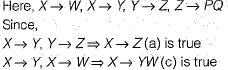Since there is no FD’s for which W functionally depends on other attributes.

QUESTION: 4

For a database relation R(a, b, c, d) where the domains of a, b, c and d include only atomic values, only the following functional dependencies and those that can be inferred from them hold.
a → c
b→d
The relation is in

Solution:

Since for Database relation R(a, b, c, d) all values are atomic and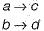Here neither of a, b, c, d are primary key.
The primary key is 'ab‘ prime attributes = {a, b} here part of key (a in a → c and in b in b → d) is dependent on non key hence there is a partial dependency and the relation is not in 2 NF.
Hence (a) is the correct option

QUESTION: 5

Third normal form is inadequate in situations where the relation

Solution:

Third normal form is considered adequate for relational database design, it is inadequate in all situations with the relation having multiple, composes or overlapped candidate keys.

QUESTION: 6

Entity set TRANSACTION has the attributes transaction number, date, amount. Entity set ACCOUNT has the attributes account number, customer name, balance.
Q. Which is the discriminator of the weak entity?

Solution:

We can clearly see that transaction is the weak entity with the discriminator (key for weak entity) as transaction number. This discriminator is used to uniquely identify each transaction of a particular account.

QUESTION: 7

Entity set TRANSACTION has the attributes transaction number, date, amount. Entity set ACCOUNT has the attributes account number, customer name, balance.
Q. Which is the primary key of the weak entity?

Solution:

In order to identify each transaction of any account the key of strong entity with the addition of the discriminator of weak entity can be taken. Here [Account number, transaction number] act as the primary key of weak entity. This is because weak entity has no existence without strong entity.

QUESTION: 8

If a relation scheme is in BCNF, then it is also in

Solution:

BCNF is the more restrictive compared to 3NF. 3NF is more restrictive as compared to 2NF and so on.
Hence option (d) is correct.

QUESTION: 9

For relation R(A, B, C, D, E, F) the set of FD’s is {A → C, B → D, C →  E, D → E, E → A, F → B} What is the candidate key for R?

Solution:

According to the given set of functional dependencies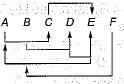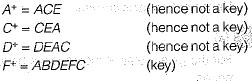QUESTION: 10

A set of FDs is given for a relation R (A, B, C, D, E, F, G)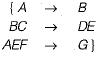What is the closure of {A, C}+ under this set?

Solution: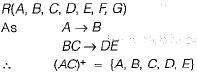Hence (d) is the correct option.